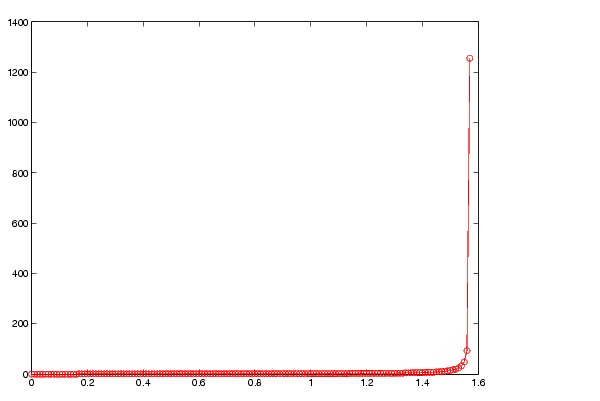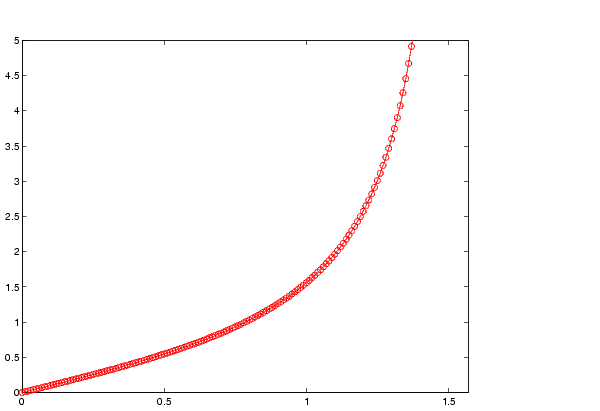MATLAB Function Referenceaxis

Axis scaling and appearance

Syntax

• ```axis([xmin xmax ymin ymax])
axis([xmin xmax ymin ymax zmin zmax cmin cmax])
v = axis

axis auto
axis manual
axis tight
axis fill

axis ij
axis xy

axis equal
axis image
axis square
```axis vis3d
```axis normal

axis off
axis on
```

axis(`axes_handles`,...)

• ```[mode,visibility,direction] = axis('state')
```

Description

`axis` manipulates commonly used axes properties. (See Algorithm section.)

```axis([xmin xmax ymin ymax]) ``` sets the limits for the x- and y-axis of the current axes.

```axis([xmin xmax ymin ymax zmin zmax cmin cmax]) ``` sets the x-, y-, and z-axis limits and the color scaling limits (see `caxis`) of the current axes.

```v = axis ``` returns a row vector containing scaling factors for the x-, y-, and z-axis. `v` has four or six components depending on whether the current axes is 2-D or 3-D, respectively. The returned values are the current axes' `XLim`, `Ylim`, and `ZLim` properties.

```axis auto ``` sets MATLAB to its default behavior of computing the current axes' limits automatically, based on the minimum and maximum values of x, y, and z data. You can restrict this automatic behavior to a specific axis. For example, `axis` `'auto` `x'` computes only the x-axis limits automatically; `axis` `'auto yz'` computes the y- and z-axis limits automatically.

```axis manual and axis(axis) ``` freezes the scaling at the current limits, so that if `hold` is `on`, subsequent plots use the same limits. This sets the `XLimMode`, `YLimMode`, and `ZLimMode` properties to `manual`.

```axis tight ``` sets the axis limits to the range of the data.

```axis fill ``` sets the axis limits and `PlotBoxAspectRatio` so that the axes fill the position rectangle. This option has an effect only if `PlotBoxAspectRatioMode` or `DataAspectRatioMode` are `manual`.

```axis ij ``` places the coordinate system origin in the upper-left corner. The i-axis is vertical, with values increasing from top to bottom. The j-axis is horizontal with values increasing from left to right.

```axis xy ``` draws the graph in the default Cartesian axes format with the coordinate system origin in the lower-left corner. The x-axis is horizontal with values increasing from left to right. The y-axis is vertical with values increasing from bottom to top.

```axis equal ``` sets the aspect ratio so that the data units are the same in every direction. The aspect ratio of the x-, y-, and z-axis is adjusted automatically according to the range of data units in the x, y, and z directions.

```axis image ``` is the same as `axis equal` except that the plot box fits tightly around the data.

```axis square ``` makes the current axes region square (or cubed when three-dimensional). MATLAB adjusts the x-axis, y-axis, and z-axis so that they have equal lengths and adjusts the increments between data units accordingly.

```axis vis3d ``` freezes aspect ratio properties to enable rotation of 3-D objects and overrides stretch-to-fill.

```axis normal ``` automatically adjusts the aspect ratio of the axes and the relative scaling of the data units so that the plot fits the figures shape as best as possible.

```axis off ``` turns off all axis lines, tick marks, and labels.

```axis on ``` turns on all axis lines, tick marks, and labels.

```axis(axes_handles,...) ``` applies the `axis` command to the specified axes. For example, the following statements

• ```h1 = subplot(221);
h2 = subplot(222);
axis([h1 h2],'square')
```

set both axes to `square`.

```[mode,visibility,direction] = axis('state') ``` returns three strings indicating the current setting of axes properties:

 Output Argument Strings Returned `mode` `'auto' | 'manual'` `visibility` `'on' | 'off'` `direction` `'xy' | 'ij'`

`mode` is `auto` if `XLimMode`, `YLimMode`, and `ZLimMode` are all set to `auto`. If `XLimMode`, `YLimMode`, or `ZLimMode` is `manual`, `mode` is `manual`.

Examples

The statements

• ```x = 0:.025:pi/2;
plot(x,tan(x),'-ro')
```

use the automatic scaling of the y-axis based on `ymax = tan(1.57)`, which is well over 1000:The right figure shows a more satisfactory plot after typing

• ```axis([0  pi/2  0  5])```

Algorithm

When you specify minimum and maximum values for the x-, y-, and z-axes, `axis` sets the `XLim`, `Ylim`, and `ZLim` properties for the current axes to the respective minimum and maximum values in the argument list. Additionally, the `XLimMode`, `YLimMode`, and `ZLimMode` properties for the current axes are set to `manual`.

`axis auto` sets the current axes' `XLimMode`, `YLimMode`, and `ZLimMode` properties to '`auto'`.

`axis manual` sets the current axes' `XLimMode`, `YLimMode`, and `ZLimMode` properties to '`manual'`.

The following table shows the values of the axes properties set by `axis equal`,
`axis normal`, `axis square`, and `axis image`.

 Axes Property axis equal axis normal axis square axis tightequal `DataAspectRatio` `[1 1 1]` `not set` `not set` `[1 1 1]` `DataAspectRatioMode` `manual` `auto` `auto` `manual` `PlotBoxAspectRatio` `[3 4 4]` `not set` `[1 1 1]` `auto` `PlotBoxAspectRatioMode` `manual` `auto` `manual` `auto` `Stretch-to-fill` `disabled` `active` `disabled` `disabled`

`axes`, `get`, `grid`, `set`, `subplot`Axes Properties balance